Importance: Medium ✭✭
 Author(s): DeVos, Matt
 Subject: Graph Theory » Coloring » » Nowhere-zero flows
 Keywords: homomorphism nowhere-zero flow tension
 Prize: bottle of wine (DeVos)
 Posted by: mdevos on: March 7th, 2007
Conjecture   Letbe abelian groups and let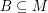andsatisfyand. If there is a homomorphism from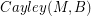to, then every graph with a B-flow has a B'-flow.

Definition:Letbe a directed graph, Let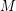be an abelian group, and letbe a subset ofsuch that. We say that a flow or a tension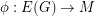is a-flow or a-tension if the range is a subset of. Ifis a-flow (-tension) ofand we reverse the direction of the edge, then we may obtain a new-flow (-tension) by changing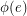to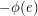. Thus, the existence of a-flow or-tension does not depend on the orientation, and we say that an undirected graph has a-flow or a-tension if some (and thus every) orientation of it admits such a map. We define the Cayley graphto be the simple graph with vertex setin which two verticesare joined by an edge if and only if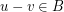.

It is well known that a graph has a-tension if and only if it has a homomorphism to. So, if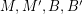are as in the conjecture and there is a homomorphism fromto, then every graph G with a-tension has a-tension. This follows from the previous sentence and the fact that the composition of two homomorphisms is another homomorphism. In essence, the above conjecture states that the same equivalence should hold true for flows.

Ifand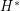are directed planar dual graphs (each edge ofcrosses left to right over the corresponding edge of), then a map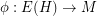is a tension if and only if the dual map(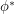is given by the rule) is a flow of. Thus, planar duality exchanges flows and tensions. For two undirected planar dual graphs,andwe have that G has a-flow if and only ifhas a-tension. It follows from this duality and the observation from the previous paragraph, that the above conjecture is true for planar graphs.

This conjecture is also known in the special case whenand. In this case,andare the complete graphs onandvertices respectively, so there is a homomorphism fromtoif and only ifis greater than or equal to. Thus, in this case the conjecture is equivalent to the assertion that every graph with a nowhere-zero-flow also has a nowhere-zero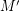-flow ifis at least. This statement is true by a result of Tutte.